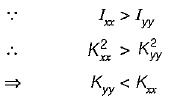Courses

# Test: Struts & Columns - 1

## 10 Questions MCQ Test Strength of Materials (SOM) | Test: Struts & Columns - 1

Description
This mock test of Test: Struts & Columns - 1 for Mechanical Engineering helps you for every Mechanical Engineering entrance exam. This contains 10 Multiple Choice Questions for Mechanical Engineering Test: Struts & Columns - 1 (mcq) to study with solutions a complete question bank. The solved questions answers in this Test: Struts & Columns - 1 quiz give you a good mix of easy questions and tough questions. Mechanical Engineering students definitely take this Test: Struts & Columns - 1 exercise for a better result in the exam. You can find other Test: Struts & Columns - 1 extra questions, long questions & short questions for Mechanical Engineering on EduRev as well by searching above.
QUESTION: 1

### Slenderness ratio has dimensions of

Solution:

Slenderness ratio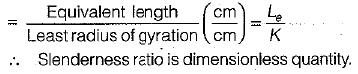QUESTION: 2

### Consider a column of aluminium alloy having modulus of elasticity 70 x 105 N/cm2 and a yield strength of 50 x 102 N/cm2. The least value of slenderness ratio will be closer to

Solution: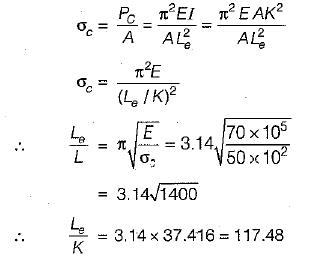QUESTION: 3

### Two steel struts have the same cross-sectional areas. One is a solid one and the other is a hollow with internal diameter three-fourth of the external diameter. The ratio of the strength of the solid steel strut to that of the hollow one is

Solution: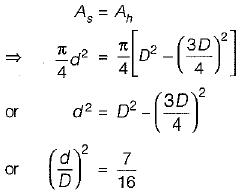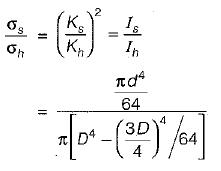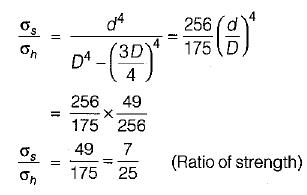QUESTION: 4

All of the following statements about kern are correct, except

Solution:

The kern of a rectangular section is diamond shaped.

QUESTION: 5

The ratio of the core of a circular section to the area of circular section when used as a short column is

Solution: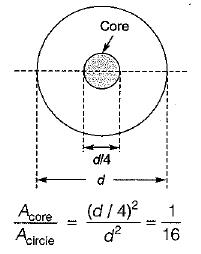QUESTION: 6

Euler’s formula gives 5 to 10% error in crippling load as compared to experimental results in practice due to

Solution:
QUESTION: 7

The ratio of critical loads a column can carry when it end conditions are best and worst is

Solution: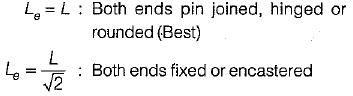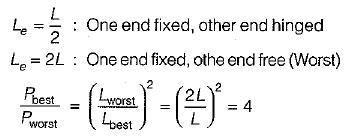QUESTION: 8

The column of length 240 cm, area of cross- section 20 cm 2, moment of inertia 1XX = 720 cm4 and Iyy = 80 cm4 is subject to buckling load. The data corresponds to slenderness ratio

Solution: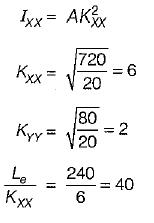and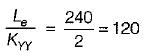Slenderness ratio is the ratio of length and least radius of gyration

∴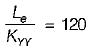QUESTION: 9

The slenderness of a column is zero when

Solution:
QUESTION: 10

A column of rectangular cross-section (IXX > IYY) is subject to an axial load. What is the axis about which the column will have atendency to buckle?

Solution:

Buckling tendency is always along least radius of gyration.